# RD Sharma Class 8 Solutions Chapter 20 Mensuration I Ex 20.3

In this chapter, we provide RD Sharma Class 8 Solutions Chapter 20 Mensuration Ex 20.3 for English medium students, Which will very helpful for every student in their exams. Students can download the latest RD Sharma Class 8 Solutions Chapter 20 Mensuration Ex 20.3 pdf, free RD Sharma Class 8 Solutions Chapter 20 Mensuration Ex 20.3 book pdf download. Now you will get step by step solution to each question.

### RD Sharma Solutions for Class 8 Chapter 20 Mensuration Ex 20.3Download PDF

Question 1.
Find the area of the pentagon shown in the figure if AD = 10 cm, AG = 8 cm, AH = 6 cm, AF = 5 cm and BF = 5 cm, CG = 7 cm and EH = 3Solution:
In the figure, here are three triangles and one trapezium.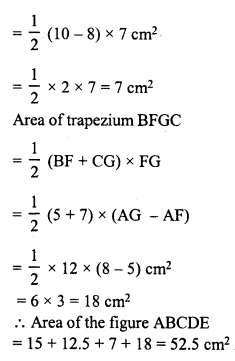Question 2.
Find the area enclosed by each of the following figures as the sum of the areas of a rectangle and a trapezium:Solution:
(i) In the figure ABCDEF,
Join CF, then, the figure consists one square and one trapezium ABCF is a square whose side = 18 cm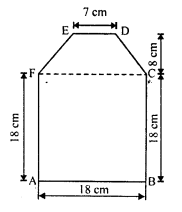Area of the square = 18 x 18 cm² = 324 cm²
Area of trapezium FCDE = 12 (CF + ED) x 8 cm²
= 12 (18 + 7) x 8
= 12 x 25 x 8 cm²
= 100 cm²
Total area of fig. ABCDEF = 324 + 100 = 424 cm²
(ii) In the figure ABCDEF,
Join BE.
The figure consists of one rectangle BCDE and one trapezium ABEFArea of rectangle BCDE = BC x CD = 20 x 15 = 300 cm²
Area of trapezium ABEF,
= 12 (BE + AF) x height
= 12 (15 + 6) x 8 cm²
= 12 x 21 x 8 cm²
= 84 cm²
Area of the figure ABCDEF = 300 + 84 = 384 cm²
(iii) In the figure ABCDEFGH,HC= AB = EF = 6 cm
AH = BC = 4 cm
DE = GF = 5 cm
Join HC.
In right ∆CDE,
ED² = CD² + CE²
⇒ (5)² = (4)² + (CE)²
⇒ 25 = 16 + (CE)²
⇒ (CE)² = 25 – 16 = 9 = (3)²
CE = 3 cm
The figure consist a rectangle and a trapezium
Area of rectangle ABCH = AB x BC = 6 x 4 = 24 cm²
Area of trapezium GDEF,
= 12 (GD + EF) x CE 1
= 12 (GH + HC + CD + EF) x CE
= 12 (4 + 6 + 4 + 6) x 3 cm²
= 12 x 20 x 3 cm²
= 30 cm²
Total area of the figure ABCDEFGH = 24 + 30 = 54 cm²

Question 3.
There is a pentagonal shaped park as shown in the figure. Jyoti and Kavita divided it in two different ways.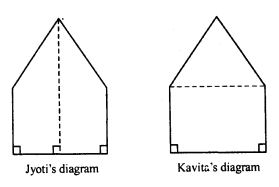Find the area of this park using both ways. Can you suggest some another way of finding its area ?
Solution:
In first case, the figure ABCDE is divided into 2 trapezium of equal area.
Now area of trapezium DFBC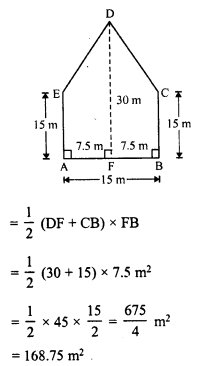Total area of the pentagon ABCDE = 2 x 168.75 = 337.5 m²
In second case, the figure ABCDE is divided into two parts, namely one square and other triangle.Total area of pentagon ABCDE = 225 + 112.5 = 337.5 m²

Question 4.
Find the area of the following polygon, if AL = 10 cm, AM = 20 cm, AN = 50 cm, AO = 60 cm and AD = 90 cm.Solution:
In the figure ABCDEF,
BL = 30 cm
AO = 60 cm
CN = 40 cm
AN = 50 cm
EO = 60 cm
AM = 20 cm
FM = 20 cm
AL = 10 cm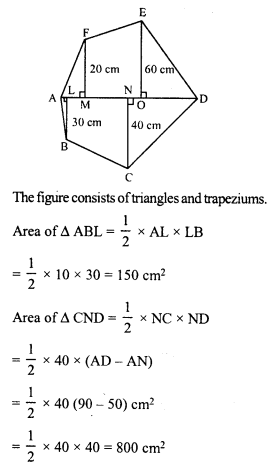Area of ABCDEF = (150 + 800 + 900 + 200 + 1400 + 1600) cm² = 5050 cm²

Question 5.
Find the area of the following regular hexagon:Solution:
In the regular hexagon MNOPQR There are two triangles and one rectangle.
Join MQ, MO and RP
NQ = 23 cm,
NA = BQ = 102 = 5 cm
MR = OP = 13 cmIn right ∆BDQ,
PQ² = BQ² + BP²
⇒ (13)² = (5)² + BP²
⇒ 169 = 25 + BP²
⇒ BP² = 169 – 25 = 144 = (12)²
BP = 12 cm
PR = MO = 2 x 12 = 24 cm
Now area of rectangle RPOM = RP x PO = 24 x 13 = 312 cm²
Area of ∆PRQ = 12 x PR x BQ
= 12 x 24 x 5 = 60 cm²
Similarly area ∆MON = 60 cm²
Area of the hexagon MNOPQR = 312 + 60 + 60 = 432 cm²

All Chapter RD Sharma Solutions For Class 8 Maths

—————————————————————————–

All Subject NCERT Exemplar Problems Solutions For Class 8

All Subject NCERT Solutions For Class 8

*************************************************

I think you got complete solutions for this chapter. If You have any queries regarding this chapter, please comment on the below section our subject teacher will answer you. We tried our best to give complete solutions so you got good marks in your exam.

If these solutions have helped you, you can also share rdsharmasolutions.in to your friends.